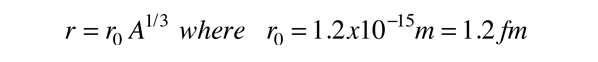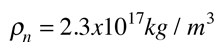# Some Nuclear Units

Nuclear energies are very high compared to atomic processes, and need larger units. The most commonly used unit is the MeV.

1 electron volt = 1eV = 1.6 x 10-19 joules
1 MeV = 106 eV; 1 GeV = 109 eV; 1 TeV = 1012 eV

However, the nuclear sizes are quite small and need smaller units:

Atomic sizes are on the order of 0.1 nm = 1 Angstrom = 10-10 m
Nuclear sizes are on the order of femtometers which in the nuclear context are usually called fermis:

1 fm = 10-15m

Atomic masses are measured in terms of atomic mass units with the carbon-12 atom defined as having a mass of exactly 12 amu. It is also common practice to quote the rest mass energy E=m0c2 as if it were the mass. The conversion to amu is:

1 u = 1.66054 x 10-27 kg = 931.494 MeV

 Electron volt definition Energies in electron volts.
Index

 HyperPhysics***** Nuclear R Nave
Go Back

# Relative scale model of an atom and the solar system

### Do you perceive a gold ring to contain a larger fraction of solid matter than the solar system?On this scale, the nearest star would be a little over 10,000 miles away.

 Model data Nuclear size.
 How does such a compact nucleus stay together?
Index

Nuclear Structure Concepts

 HyperPhysics***** Nuclear R Nave
Go Back

# Data for Scale Model of Atom

A. GOLD ATOM
Nuclear density = 2 x 1017 kg/m3
Density of solid gold = 19.32 gm/cm3
Atomic mass = 196.97 amu (1 mole = 196.97 grams)
1 amu = 1.66 x 10-27 kg
Avogadro's number = 6.02 x 1023 atoms/mole
Calculated atomic radius = 1.3 x 10-10 m
Calculated nuclear radius = 7.3 x 10-15 m
B. SOLAR SYSTEM
Radius of Sun = 432,000 miles = 695,000 km
Radius of Earth = 3963 miles = 6376 km
Sun-Earth distance = 93 x 106 miles = 150 x 106 km
Sun-Pluto distance = 3666 x 106 miles = 5900 x 106 km

 Model sketch Nuclear size and density.
Index

 HyperPhysics***** Nuclear R Nave
Go Back

# Nuclear Size and Density

Various types of scattering experiments suggest that nuclei are roughly spherical and appear to have essentially the same density. The data are summarized in the expression called the Fermi model:where r is the radius of the nucleus of mass number A. The assumption of constant density leads to a nuclear densityFor mass number A = this gives r = x10^ m r = fermi.

The most definitive information about nuclear sizes comes from electron scattering. The comparison of calculated and experimental radii for nuclei are very sensitive to the exact onset of the overlap between the probe particle and the nuclear matter. These comparisons have made it clear that there is a "tail" where the density of nuclear matter decreases toward zero. The nucleus is not a hard sphere. Krane comments that the evidence points to a mass radius and a charge radius which agree with each other within about 0.1 fermi. Since heavy nuclei have about 50% more neutrons than protons, one might expect a mass radius larger than the charge radius. One can visualize the protons being pushed toward the outside by the proton repulsion and the neutrons being pulled inward by the neutron-proton attraction, so the observed result agrees with what one might expect with this kind of model.

 Scale model of nucleus Implications about the strong force
Index

Nuclear Structure Concepts

Reference
Krane
Ch. 3

 HyperPhysics***** Nuclear R Nave
Go Back

# Nuclear Density and the Strong Force

The fact that the nuclear density seems to be independent of the details of neutron number or proton number implies that the force between the particles is essentially the same whether they are protons or neutrons. This correlates with other evidence that the strong force is the same between any pair of nucleons.

 Model sketch Nuclear size and density.
Index

 HyperPhysics***** Nuclear R Nave
Go Back

# Nuclear Particles

Nuclei are made up of protons and neutrons bound together by the strong force. Both protons and neutrons are referred to as nucleons. The number of protons is called the atomic number and determines the chemical element. Nuclei of a given element (same atomic number) may have different numbers of neutrons and are then said to be different isotopes of the element.

 Model sketch Nuclear size and density.
Index

 HyperPhysics***** Nuclear R Nave
Go Back